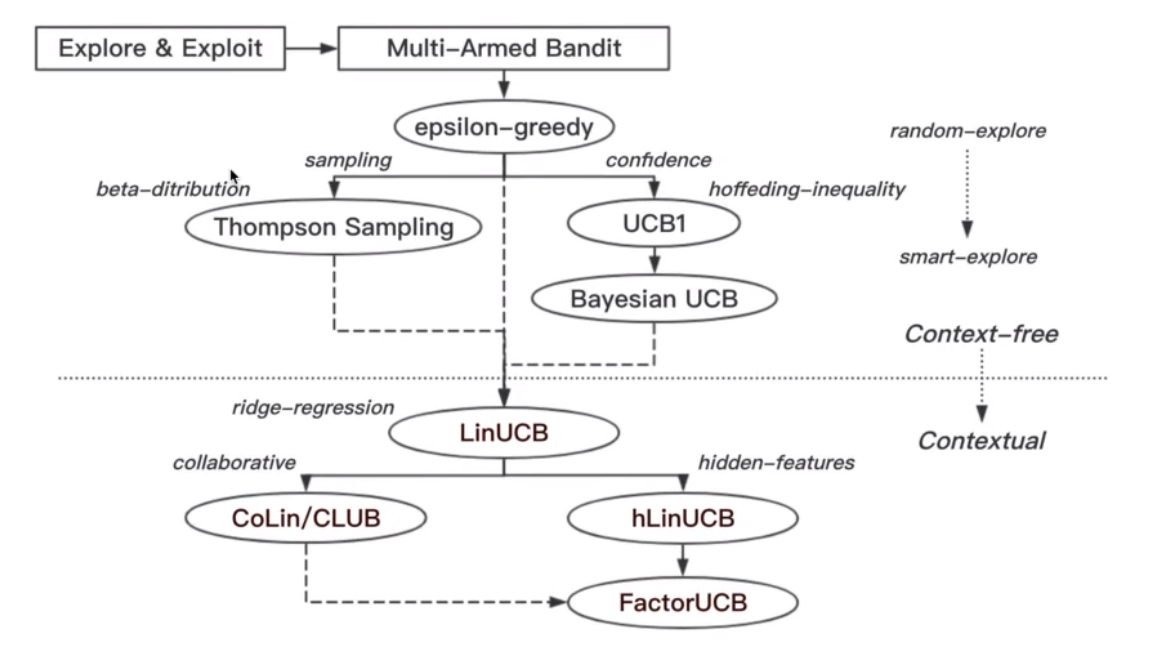# 从老虎机开始• 假设一个用户对不同类别的内容感兴趣程度不同，那么我们的推荐系统初次见到这个用户时，怎么快速地知道他对每类内容的感兴趣程度？
• 假设我们有若干广告库存，怎么知道该给每个用户展示哪个广告，从而获得最大的点击收益？是每次都挑效果最好那个么？那么新广告如何才有出头之日？
• ….

• Explore(探索):我们需要探索用户的兴趣点而且需要不断地探索新的兴趣来保证用户对系统的新鲜度,这是一个长期的,挖掘潜在价值的,成长型的需求
• Exploit(利用):当我们探索出最大价值的时候我们需要利用,这是一个贪婪的,短期的,需要奖励来反馈激励的需求

# Bandits in Recommendation

## 知识准备-累积遗憾

$$R_A(T) = E\left[\sum_{t=1}^Tr_t,a_t^*\right]-E\left[\sum_{t=1}^Tr_t,a_t\right]$$

## $\epsilon$-greedy algorithm(贪心策略)

• 使用一个我们选择的概率$\epsilon$,随机的选择一个手臂去摇老虎机(探索)
• 其他的(1-$\epsilon$)概率下,我们选择当前最赚钱的老虎机摇一下(利用)

## Thompson sampling

### Beta Distribution(Beta分布)

$$f(x;\alpha,\beta) = constant \cdot x^{\alpha-1}(1-x)^{\beta-1}=\frac {1} {B(\alpha,\beta)} x^{\alpha-1}(1-x)^{\beta-1}$$

$$var(X)=E[(X-\mu)^2]=\frac {\alpha\beta} {(\alpha+\beta)^2(\alpha+\beta+1)}$$

### 算法解释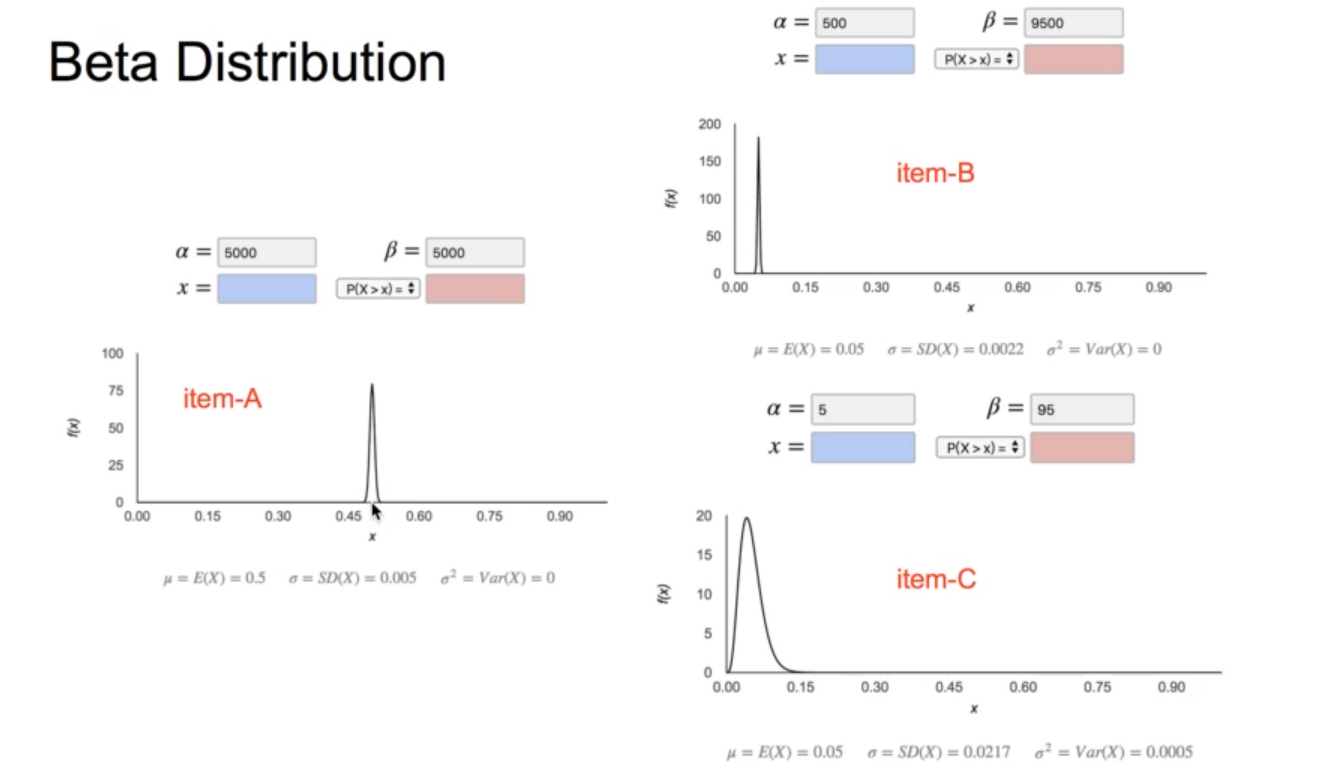item-b和item-c就是上面的摇了10000次的老虎机和摇了100次的老虎机.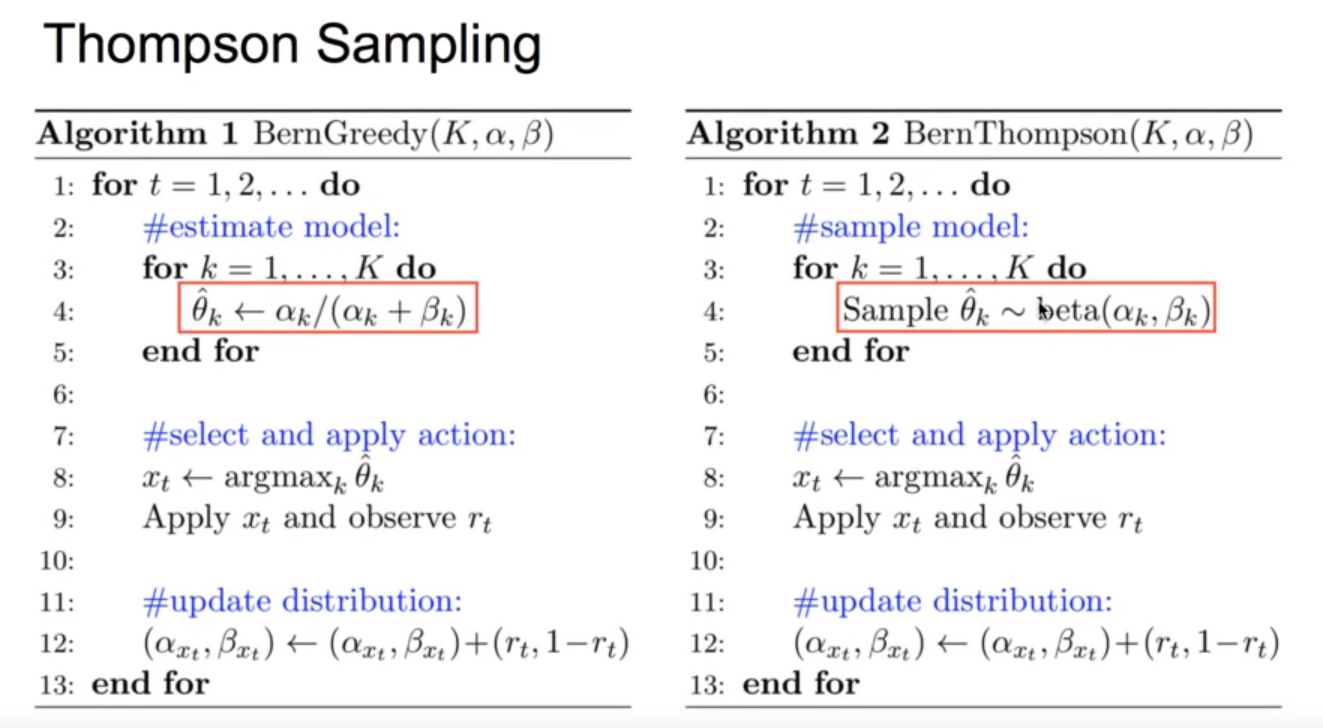## UCB:Upper Confidence Bound(置信区间上界)

$$Q(a)\leq \hat{Q_t}(a)+\hat{U_t}(a)$$

$$\hat{U_t}(a) = \sqrt{\frac {-\log p} {2N_t(a)}}$$

### Bayesian UCB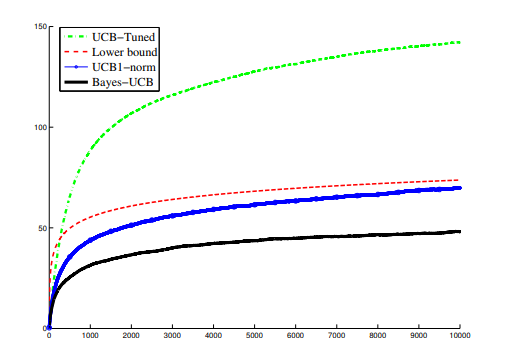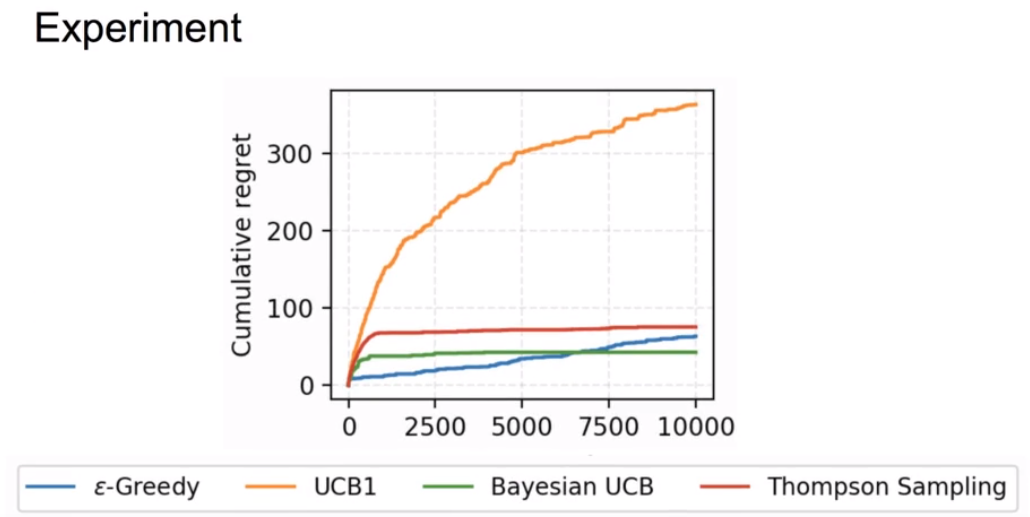## LinUCB–UCB算法的一次进化

$$E[r_{t,a}|x_{t,a}] = x_{t,a}^\top \bf {\theta}_{a\cdot}^*$$

$$loss = (C_a-D_a\theta_a)^2+\lambda\begin{Vmatrix} \theta_a \end{Vmatrix}$$

$$\theta_a = (D_a^TD_a+I)^-1D_a^Tc_a$$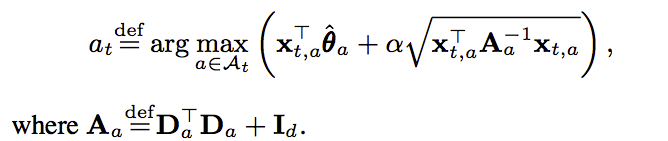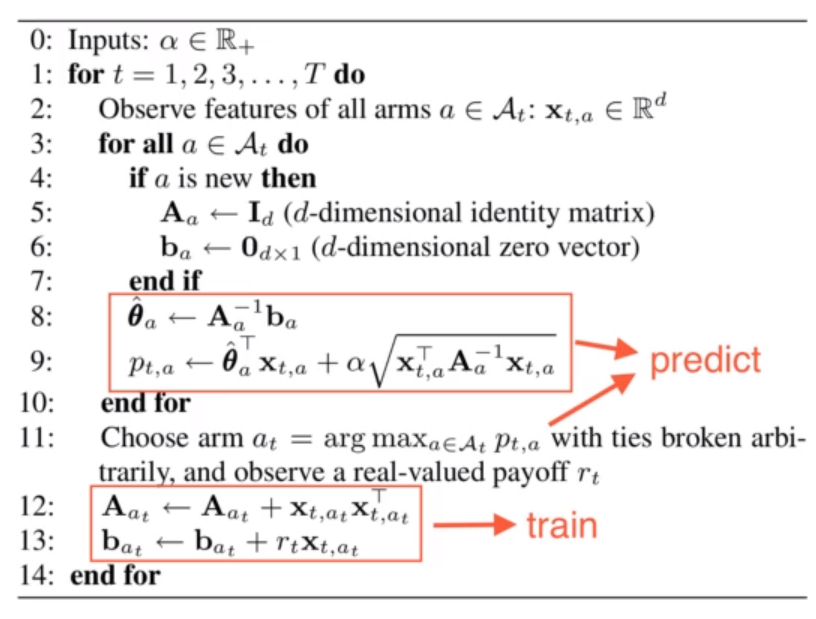### 优点

• 是上下文相关的
• 上下文特征的回报函数是线性的
• 是一个岭回归==>是有解析解的
• 服从高斯分布==>有UCB上界
• 是一个在线的算法(个人认为是:有新的数据进来不需要重新训练模型)

## 优化方向

• CoLin:协同过滤,引入了用户之间的关系(这个还是挺重要的特征)
• hLinUCB:加入了hidden-feature(隐向量)
• FactorUCB=hLinUCB+CoLin